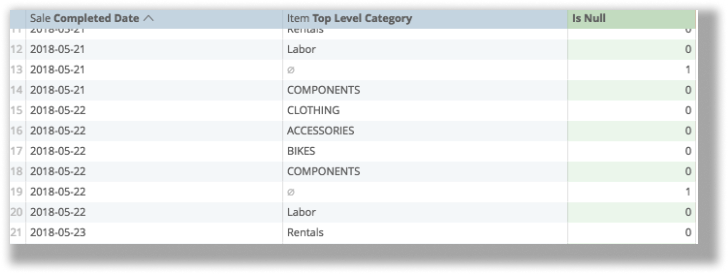# Logic functions

Lightspeed Analytics provides many functions that perform different mathematical, logical, and table-based calculations. You can find a more in-depth catalog of available functions on the Looker list of functions and operators page.

These functions are categorized in five different ways:

## And

And is used to return a result if two or more sets of criteria are met.

In this example, the date of the sale was Monday and the category of sale was Bikes.

You need at least two sets of criteria defined as IF functions. In this case, the positive and negative responses must be yes and no: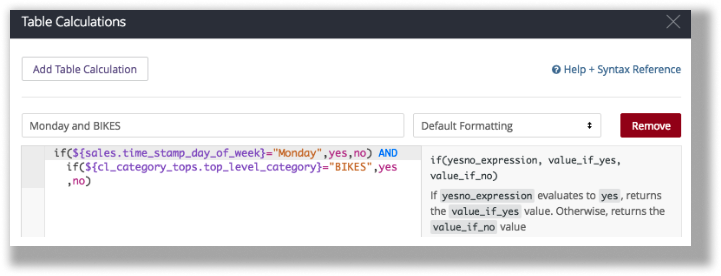The calculation looks like this:

if(\${sales.time_stamp_day_of_week}="Monday",yes,no) AND if(\${cl_category_tops.top_level_category}="BIKES",yes,no)

And if the calculation is saved properly, this is the result: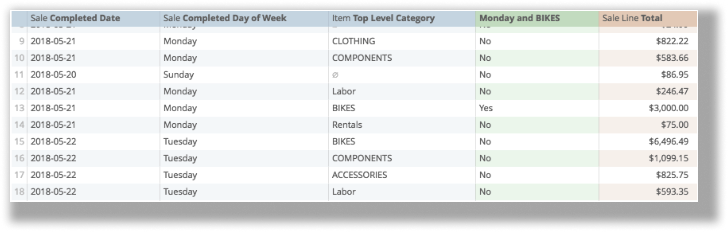## is_null

is_null returns whether a field is null. It requires one argument for the field it is searching:

For example, to find days with sales of products with no category: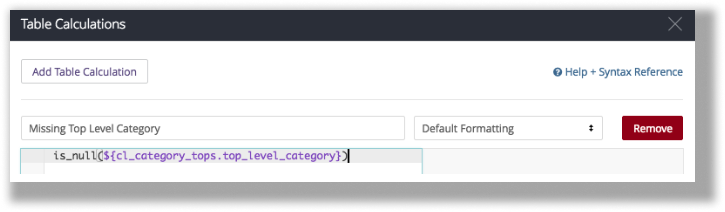The calculation looks like this:

is_null(\${cl_category_tops.top_level_category})

After you save, here is the result: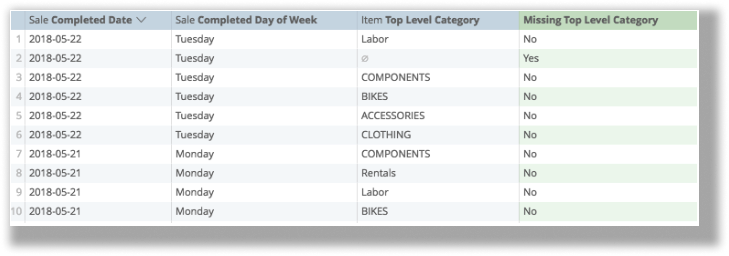## No

No is not a defined function but it is a response to logical calculations (such as is_null), which can be used in compound calculations.

In this example, Analytics finds instances of categories that are null. If they are not, Analytics returns a 1, and if they are, returns a 0: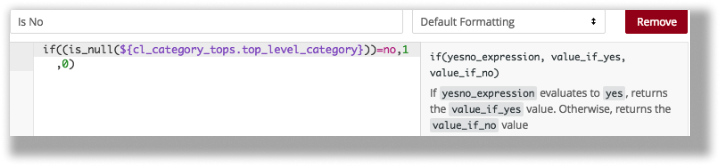The calculation looks like this:

if((is_null(\${cl_category_tops.top_level_category}))=no,1,0)

If the calculation is properly saved here is the result: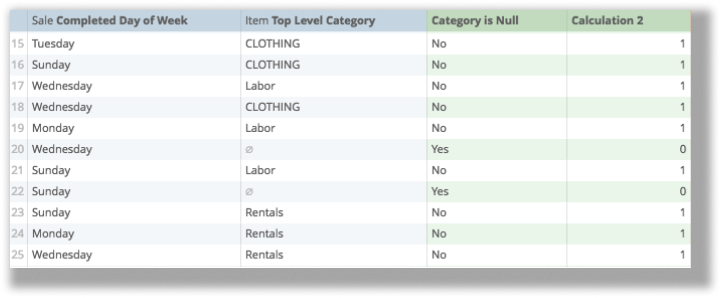## Not

To illustrate: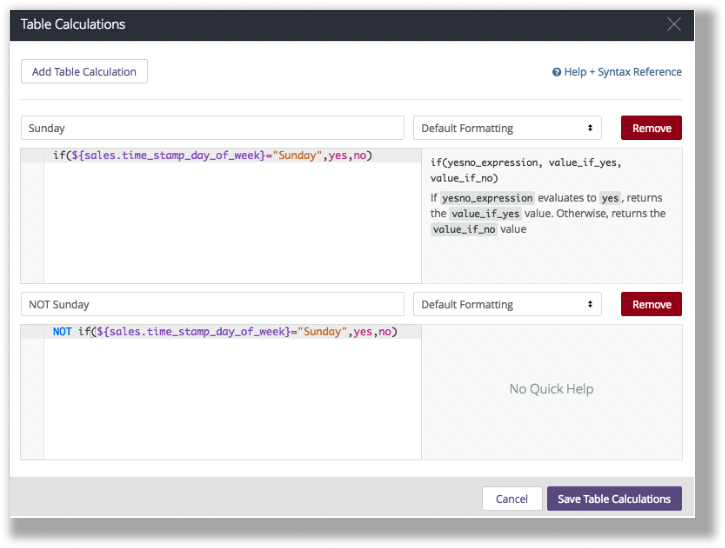The calculation looks like this:

NOT if(\${sales.time_stamp_day_of_week}="Sunday",yes,no)

If the calculation is done properly, here is the result: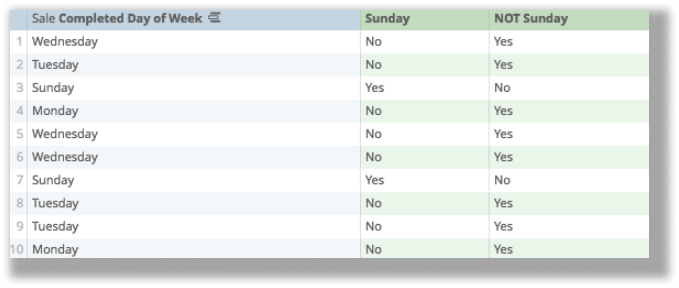## Or

Like And, Or is used to return a result if at least one of two (or more) arguments is fulfilled.

For example, in this calculation, you're looking for instances where the day of the sale was Monday, or the category of the sale was Bikes: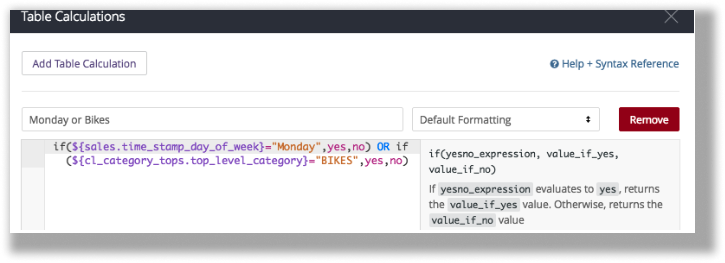The calculation looks like this:

if(\${sales.time_stamp_day_of_week}="Monday",yes,no) OR if(\${cl_category_tops.top_level_category}="BIKES",yes,no)

If the calculation is done properly, here is the result: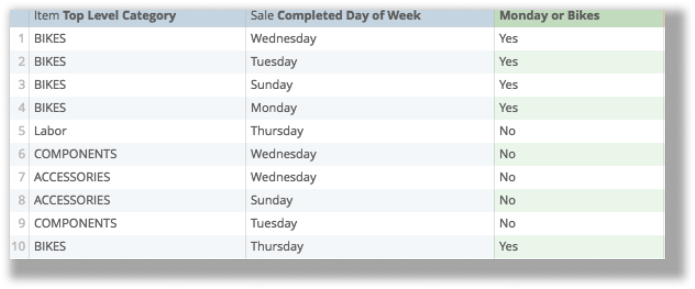## Row

Row returns the number corresponding to the row on the table. No argument is required: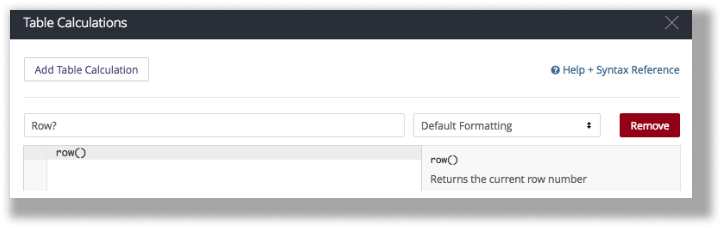The calculation looks like this:

row()

If it is prepared properly, here is the result: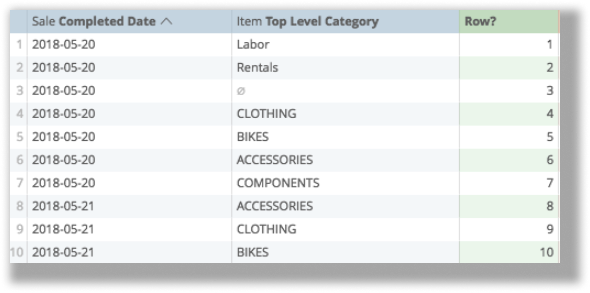## Yes

Like No, Yes is a response to logical calculations (such as is_null), which can be used in compound calculations.

In the converse example of No, search for instances where the product category is null: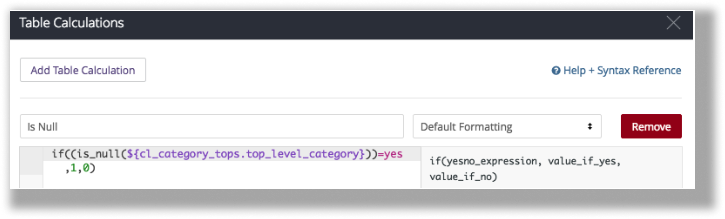The calculation looks like this:

if((is_null(\${cl_category_tops.top_level_category}))=yes,1,0)

If prepared properly, here is the result: